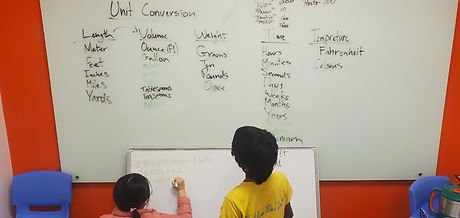Mr. Jake

Target 1​

Lesson Type:

Review

Number Operation

:

Computation

Multiply and divide fractional numbers to any place-value.

1:

Utilize the standard algorithm to divide fractions.

2:

With two fractions, find the reciprocal of the 2nd fraction, replace the ÷ with x, multiply numerators and denominators, simplify.

3:

With a whole number by a fraction, convert the whole number to an improper fraction, find the reciprocal of the 2nd fraction, replace the ÷ with x, multiply numerators and denominators, simplify.

4:

With a fraction by a whole number, convert the whole number to improper fraction, find the reciprocal of the 2nd number, replace the ÷ with x, multiply the numerators and denominators, simplify.

7th

Vocabulary:

Fraction, Arithmetic, Reciprocal

Activities:

Practiced working with fractions arithmetic, solving problems with all 4 operations

Created problems with fractions for friends to solveHome Exploration

Guiding Questions:Absent Students:

Target 2

:

1:

Describe the relationship between units that are being converted.

2:

Explain how measurement units differ or are similar.

3:

Perform arithmetic calculations to convert units of length, mass, and volume.

4:

Use ratio understandings to convert measurement units.

5:

Explain why the value of a measurement is not changing (only the unit) when using a conversion factor.

6th

Vocabulary:

Unit, Mearuement, Conversion

Activities:

Discussed what types of measurement, units, and con ersions they have worked withHome Exploration

Guiding Questions:Target 3

:

Vocabulary:

Activities:Home Exploration